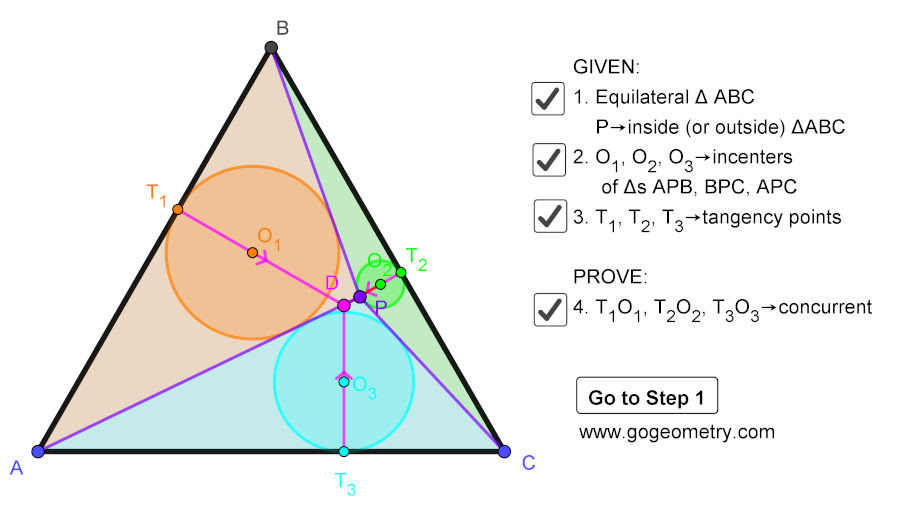## Tuesday, April 21, 2020

### Geometry Problem 1471: Equilateral Triangle, Inside/Outside Point, Incenters, Tangency Points, Concurrent Lines

Interactive step-by-step animation using GeoGebra. Post your solution in the comment box below.
Level: Mathematics Education, High School, Honors Geometry, College.

Details: Click on the figure below.#### 1 comment:

1.https://photos.app.goo.gl/4DmeeDEMfZsZ4ekS9

Let AB=BC=AC= a
Calculate PA^2-PB^2= a(P1A-P1B)
PB^2-PC^2= a(P2B-P2C)
PC^2-PA^2= a(P3C-P3A)
Add above expressions side by side and simplify ,we will have
(P1A-P1B)+ (P2B-P2C)+ (P3C-P3A)= 0…. (1)
Conversely , any 3 points P1, P2 and P3 satisfied expression (1) then the perpendiculars to the 3 sides of the equilar. Triangle will be concurred .
Let PA= u; PB=v; PC=w
We have T3C-T3A= w-u
Similarly (T1A-T1B)=u-v
And (T2B-T2C)=v-w
Add these above expression and found that the positions of T1, T2, T3 satisfy (1)
So the perpendiculars from these points to the sides will concur at a point D